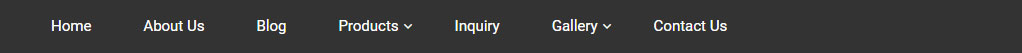Blog Detail# Page Title### How to calculate SIP returns

June 26,2021

How to Calculate SIP Returns?

There is no doubt that Systematic Investment Plans (SIP) has become one of the popular means to invest in mutual funds. After setting up an SIP, the investors invest a pre-determined amount of money at regular intervals in a specific mutual fund. You can invest in several mutual funds through SIP.

Many investors have the misconception that SIP investment is safer than onetime investment. It is just a myth and the returns on SIP investments depend on various factors such as the mutual fund category, the performance of the market and time.

In this article, we will discuss the different ways to calculate SIP returns.

Return Calculation for SIP

Calculation of SIP returns is little different from calculating returns on onetime investments. It is because SIPs are regular investments throughout the course of a period, while lump sum investment is a onetime investment.

One way that most investors calculate SIP returns to understand the growth in their SIP investments is by adding the total investment amount in the year and calculating the returns on the entire investment. However, it is not the right way to calculate the returns on SIP.

Let us take an instance for clarification. We assume that you have set up an SIP of Rs.10,000 that has given a return of 10%. In the case of SIP investments, the first SIP instalment will generate a return of Rs.1000 i.e., total 10% returns while the return on the next instalment will be Rs.916.67 and so on.

Ways to Calculate SIP returns

Here are some methods to calculate SIP returns:

Absolute Return

Absolute return is the easiest way to calculate mutual fund returns. It is also known as the point-to-point return, as the initial and present unit price (NAV) is used to calculate the returns.

Absolute return = (Present NAV – initial NAV) / initial NAV x 100

The absolute return can be used to calculate the annualised returns.

Let us assume that the NAV of a mutual fund has increased from 20 to 35 in 7 months or 210 days. In that case, the absolute return is 75%.

However, considering absolute returns to calculate SIP returns won’t be right, as the investor does not invest the total amount of SIP at one time.

Simple Annualised Return

The returns that are shown are typically yearly returns of an investment option. But what if your holding period is less than 12 months? In that case, you can annualise the returns.

Simple Annualised Return: ((1 + Absolute Rate of Return) ^ (365/number of days)) – 1

If we take the example where the absolute return was 75%, the annualised return will be

= {(1 + 75%) ^ (365/210)} – 1 = 164%

XIRR Method

XIRR is the most widely used method to calculate SIP returns as it considers the different cash flows to calculate the internal rate of return.

You can use the XIRR formula in excel to calculate the SIP returns.

XIRR formula in excel is: XIRR (value, dates, guess)

Conclusion

Calculation on SIP is little different from return calculations on onetime investment, as the SIPs are regular investments and the investment amount may vary. XIRR is the best way to calculate SIP returns. You can also use a SIP calculator to check out your SIP returns.

This blog is purely for educational purposes and not to be treated as personal advice. Mutual fund investments are subject to market risks, read all scheme-related documents carefully.

Tags :

All Blog